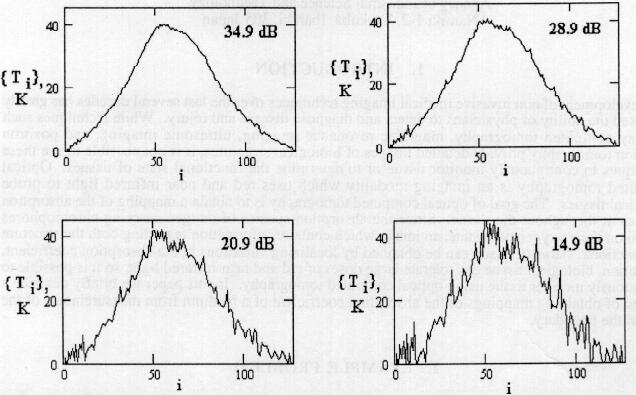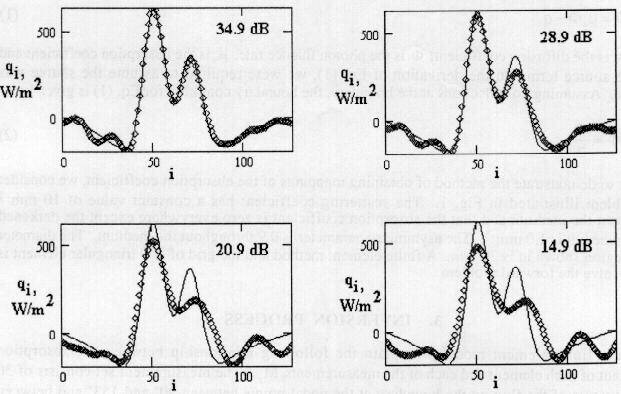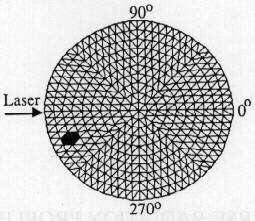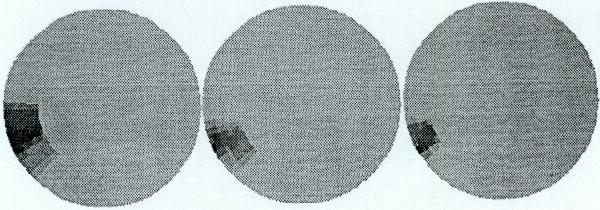### SESSION 10

#### INVERSE PROBLEMS OF RADIATIVE TRANSFER IN ABSORBING, EMITTING AND SCATTERING MEDIA

M.N. Özisik and J.C. Bokar
North Carolina State University
Mechanical and Aerospace Department
Raleigh, NC 27695-7910 U.S.A.

This work reviews and summarizes the current state-of-the-art techniques utilizing the Levenberg-Marquardt method, conjugate gradient method and a combination of both for solving inverse problems in radiative transfer. Illustrations are given in order to show the utility of each, as applied to function, parameter and property identification. We also examine the effects of measurement errors on the accuracy of estimations by inverse analysis.

In high temperature systems, radiative transfer is the fundamental mode of heat transfer. In most engineering applications the properties, the boundary conditions and the source terms in the medium are assumed to be known and the radiation flux or intensities are calculated. Such problems are referred to as the direct problem. However, if one or more of the properties or source terms unknown and measured radiation intensities are available, an inverse solution technique can be formulated in order to estimate the unknown quantity. This paper is intended to give an overview of the Levenberg-Marquardt method, conjugate gradient method and a combination of both in the solution of inverse problems of radiation transfer in participating media.

Recently, several inverse problem techniques that have come to the forefront in the radiative transfer field have their beginning in the solution of inverse problems of heat conduction. They include, among others, the conjugate gradient, Levenberg-Marquardt methods, a combination of the previous two and the Monte Carlo technique. These algorithms have been applied in such problems for the estimation of source terms and boundary conditions, properties, scattering phase function and simultaneous radiation and conduction problems. In this paper we will focus on the conjugate gradient and Levenberg-Marquardt methods for parameter and function estimation.

#### INVERSE PROBLEM TECHNIQUES

Several methods are currently being applied for solving the inverse radiation problem. Each method is best applied to a particular type of inverse problem as discussed below.

The Levenberg-Marquardt Method This technique is used solely for parameter estimation. It is a combination of a steepest descent and Newtons methods for minimization type problems. The method requires the minimization of the least squares norm functional and the solution of the sensitivity problem. The number of parameters to be solved for should not be too large as instabilities may emerge in the iteration process. The method is accurate, can handle nonlinear parameter identification, but generally needs a good starting estimate of the unknown parameters in order to achieve convergence.

The conjugate gradient method This method also uses the least squares norm and requires sensitivity coefficients as was described in the Levenberg-Marquardt technique. The conjugate gradient method can be applied to both parameter and function estimation problems. When the problem involves parameter estimation, we need only the sensitivity problem. When a function is to be estimated, however, an adjoint variable with a gradient equation must also be used in the analysis. The technique allows for initial estimates of unknown parameters or functions far from the final converged results.

The combined method For parameter estimation problems, the Levenberg-Marquardt and conjugate gradient methods may be combined in situations where a satisfactory initial guess value can not be found with the Levenberg-Marquardt method. The new algorithm uses the conjugate gradient method of solution in order to provide an initial estimate for use as the initial guess for the Levenberg-Marquardt method. The only drawback may be the extra time involved for programming.

#### EXAMPLE PROBLEMS

Problem #1 Conjugate Gradient Method for Parameter Identification
We begin with a problem that will utilize the conjugate gradient method for parameter identification for an absorbing, emitting and isotropically scattering plane parallel medium. The problem consists of the equation of transfer for isotropic scattering. The boundary conditions are with one boundary reflecting and the other transparent. The objective of the analysis is to estimate the unknown coefficients an of a volumetric source term represented as a polynomial and unknown reflectivity of the boundary surface from measured exit intensities.

Problem #2 Combined Levenberg-Marquardt and Conjugate Gradient Method (parameter)
This problem is concerned with the estimation of a temperature source term represented as a polynomial in an absorbing, emitting and isotropically scattering sphere using a combined conjugate gradient and Levenberg-Marquardt method in the sense that the converged solution of the conjugate gradient method provides the initial guess for the Levenberg-Marquardt method which is then iterated until the final converged solution is obtained.

Problem #3 Conjugate Gradient Method as Function Estimation
This problem illustrates the estimation of an unknown volumetric source term represented in functional form. The conjugate gradient method with adjoint equation is best suited for function estimation. We apply this method in order to determine an unknown temperature source term in an absorbing, emitting and anisotropically-scattering plane-parallel medium using measured exit radiation intensities.

#### INVERSE RADIATIVE ANALYSES TO DETERMINE RADIATIVE PROPERTIES USING TEMPERATURE WAVE METHOD

Andrei V. Galaktionov
Institute for High Temperatures Russian Academy of Sciences
13/19 Izhorskaya, Moscow, 127412, Russia

A universal technique based on the temperature wave method is described for nondestructive determining the spectral radiative properties at high temperatures. The temperature wave method requires pulse-periodic laser heating of a sample, and the measurements of thermal radiation on examined wavelengths, and the digital spectral analysis of laser power indicator and radiometer signals. The simplest case of non-scattering half-infinite medium with translucent mirror boundary and with small impact of radiative heat transfer on temperatures waves is considered in detail as an example. Thermal diffusivity of the sample is taken as known. The absorption coefficient on wavelength of thermal emission as well as on wavelength of excitation are to be determined from the experiment.

The sensitivity of the inverse problem to experimental errors is numerically examined without its full solving. The inverse problem is based on the approximation of the measured frequency response function of the sample by the theoretical one on several initial harmonics of basic frequency of the laser heat action. The approximation is carried out using the non-linear least squares method. The random error theory of the inverse problem is a combination of classical random process theory and well-known theory of the least square method. The sensitivity is calculated using the singular value decomposition of the design matrix. The design matrix is calculated by numerical derivation of theoretical frequency response function of the sample with respect to determined parameters.

Optimal parameters of the pulse-periodic laser heating are evaluated. Sensitivities of several kinds of the temperatures waves method are compared. The method is contrasted with known techniques of photo-thermal radiometry.

#### INVERSE RADIATIVE HEAT TRANSFER TECHNIQUE FOR HEAT FLUX RESTORATION USING OPTIMAL WIENER FILTRATION

Alexander I. Ilyinsky
Chemical Engineering Department, Ege University, 35100, Bornova, Ízmir, Turkey

The spatial distribution of energy on the treated surface should be known for better control in many applications in laser and solar technologies and material science. The increasing heat losses due to radiation, convection and conduction may change or reduce thermal efficiency of material processing. This paper presents a new technique for restoration of heat flux1 distribution for the sheet material using temperature measurements with random errors. Let us consider the thin wall with radiative heat flux distributed on the external side and cooling by convection on the internal side. Due to finite thickness of the wall conduction redistributes heat fluxes and the direct application of the Fourier law to estimation of heat fluxes on the surfaces is impossible.

The asymptotic methods have been used to reduce 3D problem into 2D formulation for thin wall and the zero approximation of 3D transient conduction equation can be written in form(1)
where x, y - coordinates on the wall, k- thermal conductivity,d- thickness of the wall, with right side term q containing difference of heat fluxes on external and internal sides of wall. the heat flux restoration algorithm is based on the spectral analysis of 2-D discrete Laplacian operator with data filtration in frequency domain using optimal Wiener filtration and quasi-Wiener filtration.

The proposed algorithm of radiative heat flux restoration can be described by following step-by-step instruction:

• The initial measured temperature data Ti,j* are converted into Fourier componentsn,m*by standard 2-D sine FFT.
• The fourier componentsn,m* are filtered by an appropriate 2-D low pass Wiener filter with frequency response wfn,m
• Calculate the corrected values of heat flux' harmonicsn,m*F by:• The recovered heat flux distribution qi,j*F is obtained by inverse 2-D sine FFT of the corrected harmonicsn,m*F
Estimations of radiative heat fluxes have been obtained for different wall thickness of the sensor and different signal to noise ration in temperature data. To study the effect of noises on the accuracy of the proposed heat flux restoration technique, the series of numerical experiments have been carried out. The initial temperature data and results of restoration are presented in Fig. 1-2. The accuracy of heat flux restoration technique has been studied both for Wiener and quasi-Wiener filtration and the simple practical criterion of selection of a regularization parameter has been proposed.

The reported results demonstrate the main features of new heat flux estimation technique; its simplicity, high rate of processing, stability and adequate accuracy and spatial resolution. The developed algorithms can be used for study of interaction of an incident radiative heat fluxes with the thin walls and creates new opportunities for radiative heat transfer measurements.Figure 1. Temperature distributions measured with errors at different averaged SNR.Figure 2. Heat flux restoration with Wiener filtration for different averaged SNR points - restored data - exact distribution

#### AN INVERSION TECHNIQUE FOR OPTICAL COMPUTED TOMOGRAPHY

Matthew R. Jones, Yukio Yamada, and Akira Tezuka
Biomechanics Division
Mechanical Engineering Laboratory
Agency of Industrial Science and Technology
Namiki 1-2, Tsukuba, Ibaraki, 305 Japan

#### 1. INTRODUCTION

The development of non invasive medical imaging techniques over the last several decades has greatly increased the ability of physicians to detect and diagnose disease and injury. While techniques such as x-ray computed tomography, magnetic resonance imaging, ultrasonic imaging, and positron emission tomography provide detailed images of biological structures, it is not possible to use these techniques to continuously monitor tissue or to determine the functional state of tissues. Optical computed tomography is an imaging modality which uses red and near infrared light to probe biological tissues. The goal of optical computed tomography is to obtain a mapping of the absorption coefficient throughout the tissue. Since the absorption spectra of oxygen carrying chromophores depend on their oxygenation state, an image which contains information regarding both the structure and functional status of tissue can be obtained by localizing variations in the absorption coefficient. In addition, biological tissue can tolerate large doses of red and near infrared light, so it is possible to continuously monitor tissue using optical computed tomography. In this paper we briefly describe a method of obtaining mappings of the absorption coefficient of a medium from measurements of the flux on the boundary.

#### 2. EXAMPLE PROBLEM

Light propagation in highly scattering, weakly absorbing medium such as biological tissue is often described using the diffusion or P1 approximation to the radiative transfer equation. The time independent photon diffusion equation is given by(1)
whereis the diffusion coefficient,is the photon fluence rate,ais the absorption coefficient and q is the source term. In the derivation of Eq. (1), we were required to assume the source was isotropic. Assuming no reflections at the boundary, the boundary condition for Eq. (1) is given by(2)
In order to demonstrate the method of obtaining mappings of the absorption coefficient, we consider the problem illustrated in Fig.1. The scattering coefficient has a constant value of 10 mm-1 throughout the medium and that the absorption coefficient is zero everywhere except the darkened region, where it is 1.0 mm-1. The asymmetry parameter is 0.9 throughout the medium. The diameter of the region shown in is 20 mm. A finite element method and the grid of 800 triangular element is used to solve the forward problem.

#### 3. INVERSION PROCESS

Using the finite element model we obtain the following relationship between the absorption coefficient of each element and each of the measurements, Mp. The measurement set consists of 30 measurements of the flux on the boundary at the nodal points between 90o and 153o and between 207o 270o.(3)Figure 1. Finite Element Model

In Eq. (3)Ai is the absorption coefficient from the previous iteration and the Jacobian matrix, J, represents the sensitivity of the pth measurements to changes in the absorption coefficient of the qth element. Assuming that the absorption coefficient is initially zero everywhere, we obtain successive approximations by inverting Eq. (3) until convergence is obtained. However, since the number of elements is much larger than the number of measurements, Eq. (3) represents a highly underdetermined system of equations if we vary theof every element. We overcome the underdetermined nature of the problem by grouping the elements into blocks and varying the absorption coefficient of each element in a block by the same amount. Unfortunately this process also greatly reduces the resolution of the mapping. We are able to increase the resolution of the mapping by using what we call the zooming method. In the zooming method we initially group all the elements into large blocks and obtain a low resolution mapping. We then concentrate the blocks in regions where inhomogeneities appear to be present, hold the absorption coefficient constant outside the region of interest, and repeat the solution process. Equation (3) is inverted using the singular value decomposition of the Jacobian matrix.

#### 4. RESULTS

Figure 2 shows how resolution of the absorption coefficient mapping is improved by using the zooming method. The mapping on the left hand side was obtained when all the elements were grouped into 36 blocks which contain between 16 and 27 elements each. The mapping in the middle was obtained using 30 blocks containing 2 or 3 elements each. The mapping on the right hand side was obtained using 21 blocks which contain only one element each.Figure 2. Absorption Coefficient Mappings